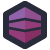As developers, we often have to engage with management who doesn't have a clue what it is we do, or how. Even if that manager was technical once, their technical background is frequently out of date, and their spirit has been sapped by the endless meetings and politics that being a manager entails. And it's often these managers who have some degree of control over where our career is going to progress, so we need to make them happy.

Which means… <clickbait-voice>LEVEL UP YOUR CAREER WITH THIS ONE SIMPLE TRICK!</clickbait-voice>. You need to make managers happy, and if there's one thing that makes managers happy, it's dashboards. Take something complicated and multivariate, and boil it down to a simple system. Traffic lights are always a favorite: green is good, red is bad, yellow is also bad.

It sounds stupid, because it is, but one of the applications that got me the most accolades was a dashboard application. It was an absolute trainwreck of code that slurped data from a dozen different silos and munged it together via a process the customer was always tweaking, and turned the complicated mathematics of how much wasteage there was in an industrial process into a simple traffic light icon. Upper managers used it and loved it, because that little glowing green light gave them all the security they needed, and when one of those lights went yellow or worse, red, they could swoop in and do management until the light turned green again.

Well, Kaspar also supports a dashboard application. It also slurps giant piles of data from a variety of sources, and it tries to turn some key metrics into simple letter grades- "A" through "E".

This particular query is about 400 lines of subqueries connected via `LEFT JOIN`. The whole thing is messy in the way that only giant SQL queries that are trying to restructure and reshape data in extreme ways can be. That's not truly a WTF, but several of these subqueries do something… special.

``````(select
Rating_mangler =
case
WHEN  VALUE = '' THEN ''
WHEN a_id in (SELECT actor.id
FROM actor, f_cache, f_math
WHERE  f_cache.a_id=actor.id AND f_math.name IN ('L43A0', 'L43A1', 'L43A2A3', 'L33OEKO') AND f_cache.formula_id=f_math.id
AND filter = '' AND treaarsregle=1 AND e_count_value=0) THEN ''
WHEN  VALUE < '1.9999999999' THEN 'E'
WHEN  VALUE >= '2' and  VALUE< '2.99999999' THEN 'D'
WHEN  VALUE >='3' and  VALUE < '3.999999999' THEN 'C'
WHEN  VALUE  >='4' and  VALUE < '4.999999999' THEN 'B'
ELSE 'A'
END, a_id
From f_cache, f_math
where formula_id=f_math.id
and f_math.name in ('L_V_mangler_p')
and filter = '' and treAarsregle=1 and pricetype=2 and e_count_hp=0) as Rating_mangler
``````

Specifically, I want to highlight the chain of `WHEN` clauses in that case. We're translating ranges into letter grades, but those ranges are stored as text. We're doing range queries on on text: `WHEN VALUE >= '2' and VALUE< '2.99999999' THEN 'D'`.

Now, this has some interesting effects. First, if the `VALUE` is "20", that's a "D". A value of "100" is going to be an "E". And since it's text, "WTF" is also going to be an "A".

We can hope that input validation at least keeps most of those values out. But this pattern repeats. There are other subqueries in this whole chain. Like:

``````(select
Rating_Arbejdsulykker =
case
WHEN  VALUE = '' THEN ''
WHEN  VALUE < '1.9999999999' THEN 'E'
WHEN  VALUE >= '2' and  VALUE< '2.99999999' THEN 'D'
WHEN  VALUE >='3' and  VALUE < '3.999999999' THEN 'C'
WHEN  VALUE  >='4' and  VALUE < '4.999999999' THEN 'B'
ELSE 'A'
END, a_id
From f_cache, f_math
where formula_id=f_math.id
and f_math.name in ('L_V_ulykker_p')
and filter = '' and treAarsregle=1 and pricetype=2 and e_count_hp=0) as Rating_Arbejdsulykker
``````

And yet again, but for bonus points, we do it using a totally different way of describing the range:

``````(select
Rating_kundetilfredshed =
case
WHEN a_id in (SELECT actor.id
FROM actor, f_cache, f_math
WHERE  f_cache.a_id=actor.id AND f_math.name IN ('L153', 'L153LOYAL') AND f_cache.formula_id=f_math.id
AND filter = '' AND treaarsregle=1 AND e_count_value=0) THEN ''
WHEN  VALUE = '' THEN ''
WHEN  VALUE = '1' THEN 'E'
WHEN  VALUE >= '1.000001' and  VALUE<= '2.00001' THEN 'D'
WHEN  VALUE >='2.00001' and  VALUE <= '3.00001' THEN 'C'
WHEN  VALUE  >'3.00001' and  VALUE <= '4.00001' THEN 'B'
ELSE 'A'
END, a_id
From f_cache, f_math
where formula_id=f_math.id
and f_math.name in ('L153_AVG')
and filter = '' and treAarsregle=1 and pricetype=2 and e_count_hp=0) as Rating_kundetilfredshed
``````

Unlike the others, this one would score values less than "1" as an "A". Which who knows, maybe values less than one are prevented by input validation. Of course, if they stored numbers as numbers then we could compare them as numbers, and all of this would work correctly without having to take it on faith that the data in the database is good.[Advertisement] Otter - Provision your servers automatically without ever needing to log-in to a command prompt. Get started today!